The OpenFOAM Foundation
rhoConst< Specie > Class Template Reference

RhoConst (rho = const) of state. More...

Inheritance diagram for rhoConst< Specie >: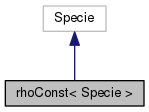[legend]
Collaboration diagram for rhoConst< Specie >:[legend]

## Public Member Functions

rhoConst (const Specie &sp, const scalar rho)
Construct from components. More...

rhoConst (Istream &)
Construct from Istream. More...

rhoConst (const dictionary &dict)
Construct from dictionary. More...

rhoConst (const word &name, const rhoConst &)
Construct as named copy. More...

autoPtr< rhoConstclone () const
Construct and return a clone. More...

scalar rho (scalar p, scalar T) const
Return density [kg/m^3]. More...

scalar h (const scalar p, const scalar T) const
Return enthalpy departure [J/kmol]. More...

scalar cp (scalar p, scalar T) const
Return cp departure [J/(kmol K]. More...

scalar s (const scalar p, const scalar T) const
Return entropy [J/(kmol K)]. More...

scalar psi (scalar p, scalar T) const
Return compressibility rho/p [s^2/m^2]. More...

scalar Z (scalar p, scalar T) const
Return compression factor []. More...

scalar cpMcv (scalar p, scalar T) const
Return (cp - cv) [J/(kmol K]. More...

void write (Ostream &os) const
Write to Ostream. More...

void operator+= (const rhoConst &)

void operator-= (const rhoConst &)

void operator*= (const scalar)

## Static Public Member Functions

static autoPtr< rhoConstNew (Istream &is)

static word typeName ()
Return the instantiated type name. More...

## Static Public Attributes

static const bool incompressible = true
Is the equation of state is incompressible i.e. rho != f(p) More...

static const bool isochoric = true
Is the equation of state is isochoric i.e. rho = const. More...

## Friends

rhoConst operator+ (const rhoConst &, const rhoConst &)

rhoConst operator- (const rhoConst &, const rhoConst &)

rhoConst operator* (const scalar s, const rhoConst &)

rhoConst operator== (const rhoConst &, const rhoConst &)

Ostreamoperator (Ostream &, const rhoConst &)

## Detailed Description

### template<class Specie> class Foam::rhoConst< Specie >

RhoConst (rho = const) of state.

Source files

Definition at line 47 of file rhoConst.H.

## Constructor & Destructor Documentation

 rhoConst ( const Specie & sp, const scalar rho )
inline

Construct from components.

Definition at line 32 of file rhoConstI.H.

References Foam::name().

Here is the call graph for this function: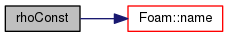rhoConst ( Istream & is )

Construct from Istream.

Definition at line 32 of file rhoConst.C.

References IOstream::check().

Here is the call graph for this function: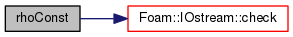rhoConst ( const dictionary & dict )

Construct from dictionary.

Definition at line 42 of file rhoConst.C.

 rhoConst ( const word & name, const rhoConst< Specie > & )
inline

Construct as named copy.

## Member Function Documentation

 Foam::autoPtr< Foam::rhoConst< Specie > > clone ( ) const
inline

Construct and return a clone.

Definition at line 58 of file rhoConstI.H.

 Foam::autoPtr< Foam::rhoConst< Specie > > New ( Istream & is )
inlinestatic

Definition at line 66 of file rhoConstI.H.

 static word typeName ( )
inlinestatic

Return the instantiated type name.

Definition at line 126 of file rhoConst.H.

 Foam::scalar rho ( scalar p, scalar T ) const
inline

Return density [kg/m^3].

Definition at line 75 of file rhoConstI.H.

 Foam::scalar h ( const scalar p, const scalar T ) const
inline

Return enthalpy departure [J/kmol].

Definition at line 82 of file rhoConstI.H.

 Foam::scalar cp ( scalar p, scalar T ) const
inline

Return cp departure [J/(kmol K].

Definition at line 89 of file rhoConstI.H.

 Foam::scalar s ( const scalar p, const scalar T ) const
inline

Return entropy [J/(kmol K)].

Definition at line 96 of file rhoConstI.H.

 Foam::scalar psi ( scalar p, scalar T ) const
inline

Return compressibility rho/p [s^2/m^2].

Definition at line 103 of file rhoConstI.H.

 Foam::scalar Z ( scalar p, scalar T ) const
inline

Return compression factor [].

Definition at line 110 of file rhoConstI.H.

 Foam::scalar cpMcv ( scalar p, scalar T ) const
inline

Return (cp - cv) [J/(kmol K].

Definition at line 117 of file rhoConstI.H.

 void write ( Ostream & os ) const

Write to Ostream.

Definition at line 52 of file rhoConst.C.

References dictionary::add(), dict, dictionaryName::dictName(), Foam::indent(), token::SPACE, and write().

Here is the call graph for this function: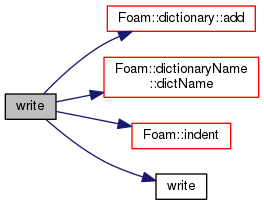void operator+= ( const rhoConst< Specie > & )
inline

Definition at line 126 of file rhoConstI.H.

 void operator-= ( const rhoConst< Specie > & )
inline

Definition at line 140 of file rhoConstI.H.

 void operator*= ( const scalar s )
inline

Definition at line 154 of file rhoConstI.H.

References s().

Here is the call graph for this function: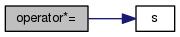## Friends And Related Function Documentation

 rhoConst operator+ ( const rhoConst< Specie > & , const rhoConst< Specie > & )
friend
 rhoConst operator- ( const rhoConst< Specie > & , const rhoConst< Specie > & )
friend
 rhoConst operator* ( const scalar s, const rhoConst< Specie > & )
friend
 rhoConst operator== ( const rhoConst< Specie > & , const rhoConst< Specie > & )
friend
 Ostream& operator ( Ostream & , const rhoConst< Specie > & )
friend

## Member Data Documentation

 const bool incompressible = true
static

Is the equation of state is incompressible i.e. rho != f(p)

Definition at line 135 of file rhoConst.H.

 const bool isochoric = true
static

Is the equation of state is isochoric i.e. rho = const.

Definition at line 138 of file rhoConst.H.

The documentation for this class was generated from the following files:
• src/thermophysicalModels/specie/equationOfState/rhoConst/rhoConst.H
• src/thermophysicalModels/specie/equationOfState/rhoConst/rhoConst.C
• src/thermophysicalModels/specie/equationOfState/rhoConst/rhoConstI.H